# Homework 7 Problem 1 Homework 7 Problem 1

• Slides: 77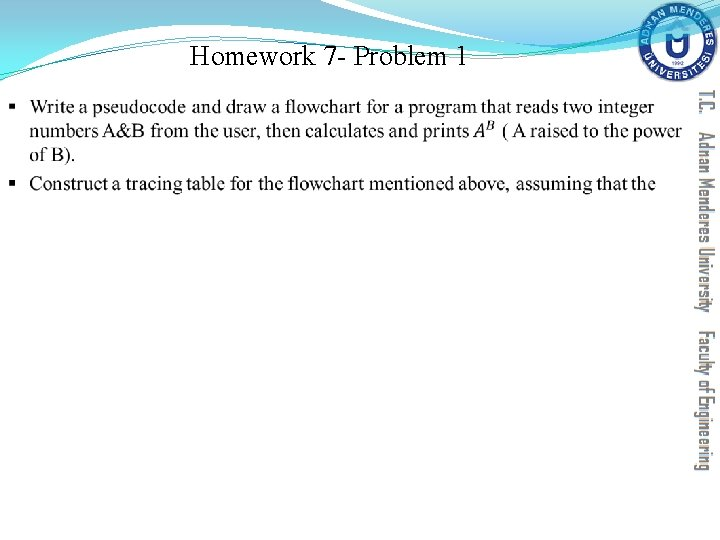Homework 7 - Problem 1 §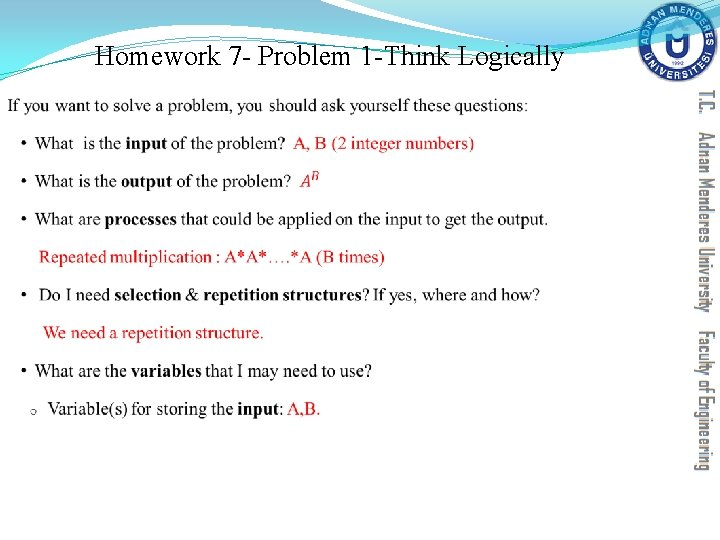Homework 7 - Problem 1 -Think Logically §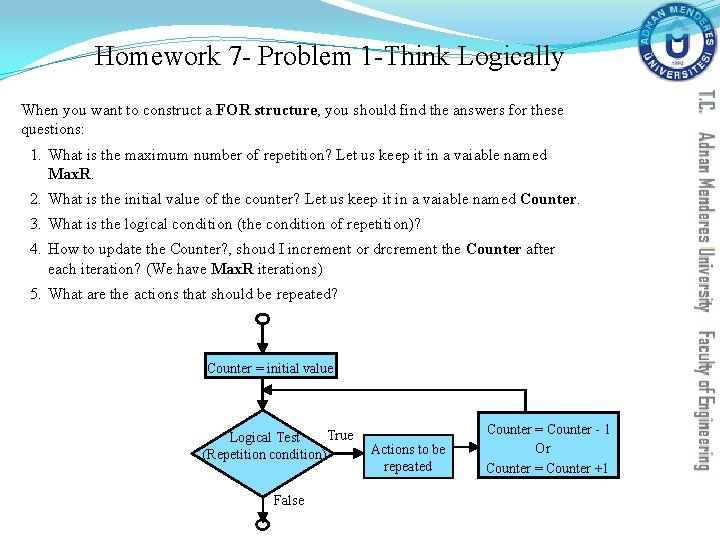Homework 7 - Problem 1 -Think Logically When you want to construct a FOR structure, you should find the answers for these questions: 1. What is the maximum number of repetition? Let us keep it in a vaiable named Max. R. 2. What is the initial value of the counter? Let us keep it in a vaiable named Counter. 3. What is the logical condition (the condition of repetition)? 4. How to update the Counter? , shoud I increment or drcrement the Counter after each iteration? (We have Max. R iterations) 5. What are the actions that should be repeated? Counter = initial value True Logical Test (Repetition condition) False Actions to be repeated Counter = Counter - 1 Or Counter = Counter +1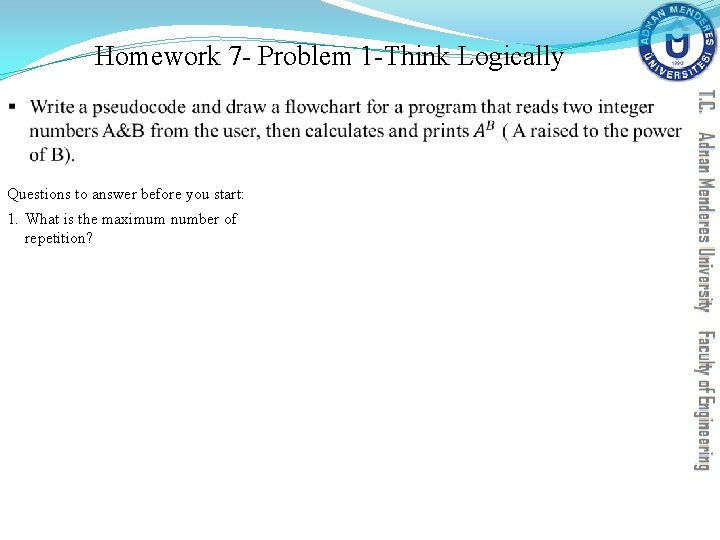Homework 7 - Problem 1 -Think Logically § Questions to answer before you start: 1. What is the maximum number of repetition?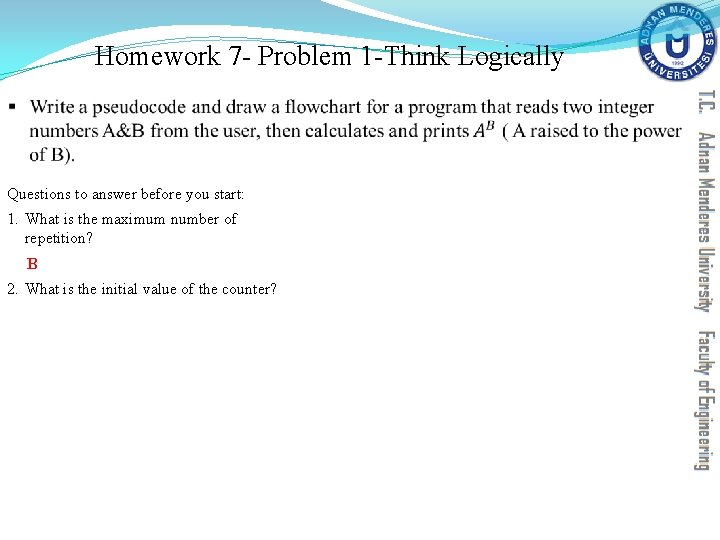Homework 7 - Problem 1 -Think Logically § Questions to answer before you start: 1. What is the maximum number of repetition? B 2. What is the initial value of the counter?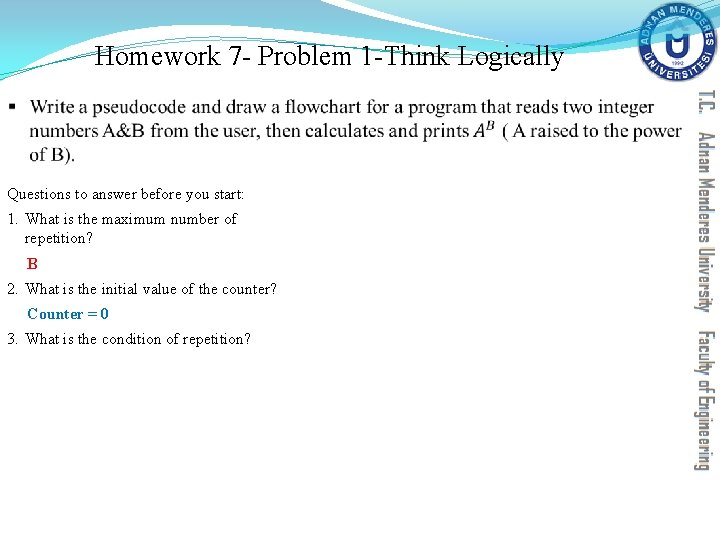Homework 7 - Problem 1 -Think Logically § Questions to answer before you start: 1. What is the maximum number of repetition? B 2. What is the initial value of the counter? Counter = 0 3. What is the condition of repetition?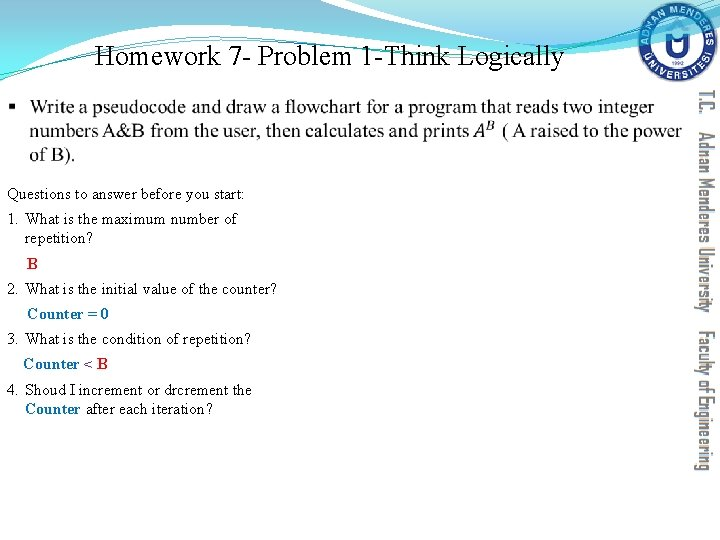Homework 7 - Problem 1 -Think Logically § Questions to answer before you start: 1. What is the maximum number of repetition? B 2. What is the initial value of the counter? Counter = 0 3. What is the condition of repetition? Counter < B 4. Shoud I increment or drcrement the Counter after each iteration?Homework 7 - Problem 1 -Think Logically § Questions to answer before you start: 1. What is the maximum number of repetition? B 2. What is the initial value of the counter? Counter = 0 3. What is the condition of repetition? Counter < B 4. Shoud I increment or drcrement the Counter after each iteration? Counter = Counter+1 5. What are the actions that should be repeated?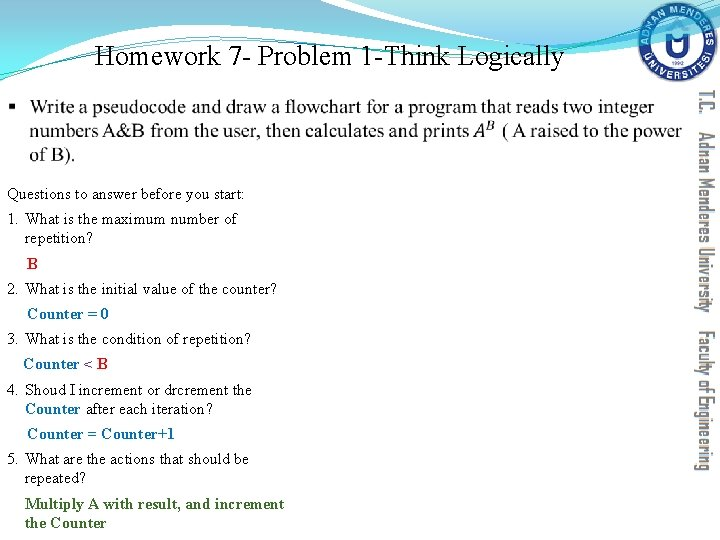Homework 7 - Problem 1 -Think Logically § Questions to answer before you start: 1. What is the maximum number of repetition? B 2. What is the initial value of the counter? Counter = 0 3. What is the condition of repetition? Counter < B 4. Shoud I increment or drcrement the Counter after each iteration? Counter = Counter+1 5. What are the actions that should be repeated? Multiply A with result, and increment the Counter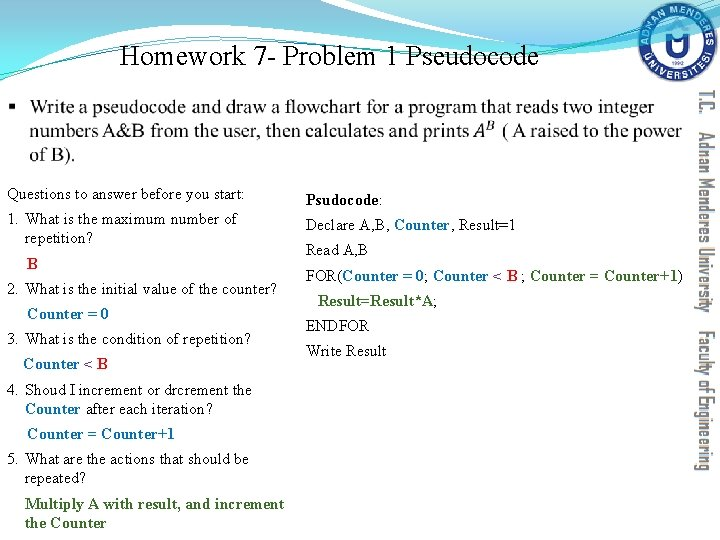Homework 7 - Problem 1 Pseudocode § Questions to answer before you start: Psudocode: 1. What is the maximum number of repetition? Declare A, B, Counter, Result=1 B 2. What is the initial value of the counter? Counter = 0 3. What is the condition of repetition? Counter < B 4. Shoud I increment or drcrement the Counter after each iteration? Counter = Counter+1 5. What are the actions that should be repeated? Multiply A with result, and increment the Counter Read A, B FOR(Counter = 0; Counter < B ; Counter = Counter+1) Result=Result*A; ENDFOR Write Result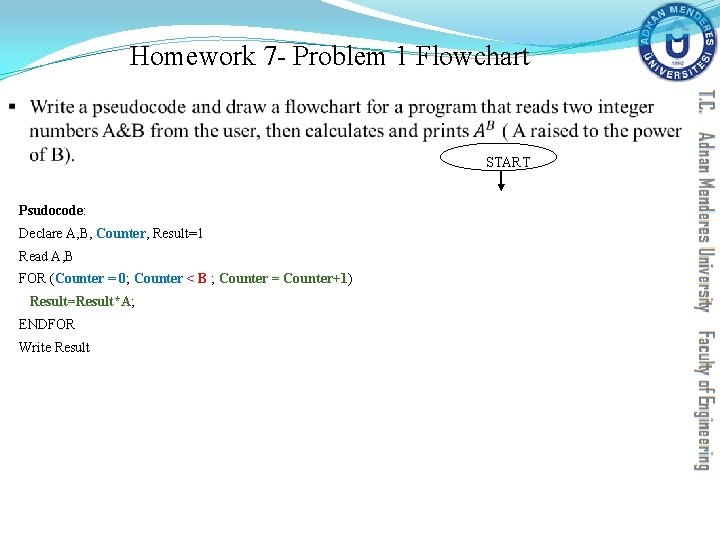Homework 7 - Problem 1 Flowchart § START Psudocode: Declare A, B, Counter, Result=1 Read A, B FOR (Counter = 0; Counter < B ; Counter = Counter+1) Result=Result*A; ENDFOR Write Result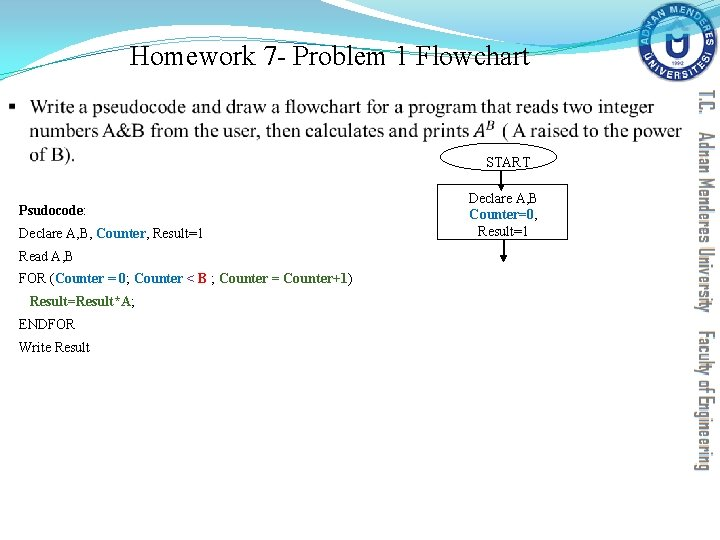Homework 7 - Problem 1 Flowchart § START Psudocode: Declare A, B, Counter, Result=1 Read A, B FOR (Counter = 0; Counter < B ; Counter = Counter+1) Result=Result*A; ENDFOR Write Result Declare A, B Counter=0, Result=1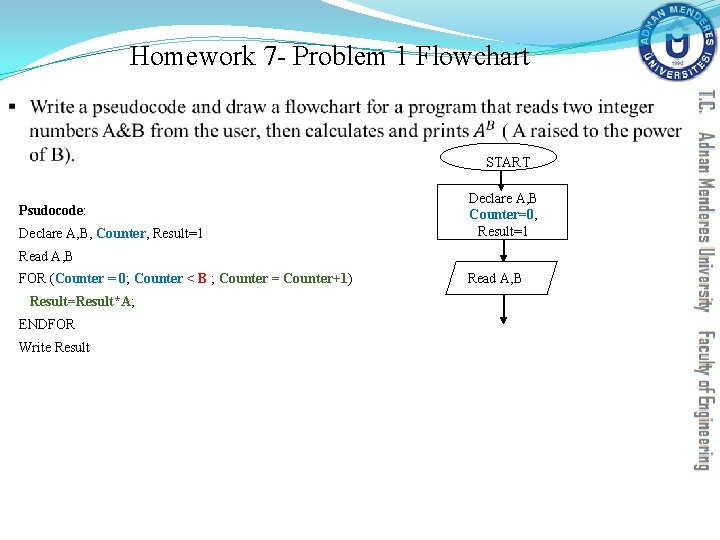Homework 7 - Problem 1 Flowchart § START Psudocode: Declare A, B, Counter, Result=1 Declare A, B Counter=0, Result=1 Read A, B FOR (Counter = 0; Counter < B ; Counter = Counter+1) Result=Result*A; ENDFOR Write Result Read A, BHomework 7 - Problem 1 Flowchart § START Psudocode: Declare A, B, Counter, Result=1 Declare A, B Counter=0, Result=1 Read A, B FOR (Counter = 0; Counter < B ; Counter = Counter+1) Read A, B Result=Result*A; ENDFOR Write Result False Logical Condition TrueHomework 7 - Problem 1 Flowchart § START Declare A, B Counter=0, Result=1 Psudocode: Declare A, B, Counter, Result=1 Read A, B FOR (Counter = 0; Counter < B ; Counter = Counter+1) Result=Result*A; ENDFOR Write Result False Counter<B TrueHomework 7 - Problem 1 Flowchart § START Declare A, B Counter=0, Result=1 Psudocode: Declare A, B, Counter, Result=1 Read A, B FOR (Counter = 0; Counter < B ; Counter = Counter+1) Result=Result*A; ENDFOR Write Result False Counter<B True Actions that should be repeated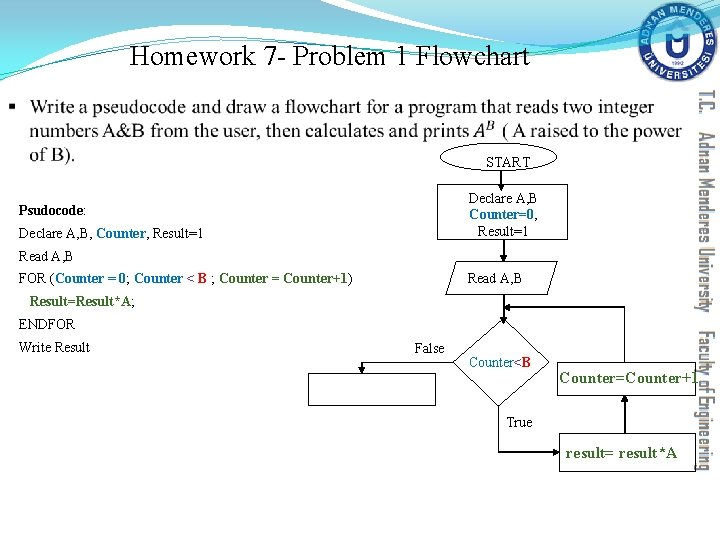Homework 7 - Problem 1 Flowchart § START Declare A, B Counter=0, Result=1 Psudocode: Declare A, B, Counter, Result=1 Read A, B FOR (Counter = 0; Counter < B ; Counter = Counter+1) Result=Result*A; ENDFOR Write Result False Counter<B Counter=Counter+1 True result= result*A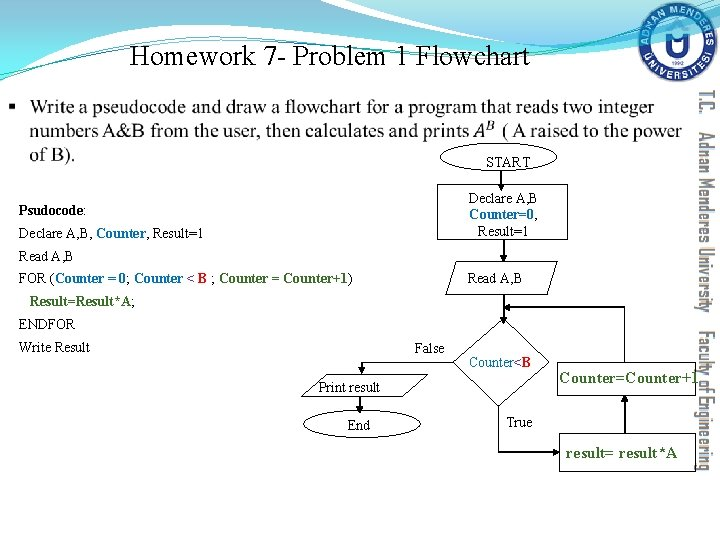Homework 7 - Problem 1 Flowchart § START Declare A, B Counter=0, Result=1 Psudocode: Declare A, B, Counter, Result=1 Read A, B FOR (Counter = 0; Counter < B ; Counter = Counter+1) Result=Result*A; ENDFOR Write Result False Counter<B Print result End Counter=Counter+1 True result= result*AHomework 7 - Problem 1 Tracing Table Construct a tracing table for the flowchart mentioned above, assuming that the user has entered A=3, B=4. START Declare A, B Counter=0, Result=1 Read A, B False Counter<B Counter=Counter+1 Print result End True result= result*A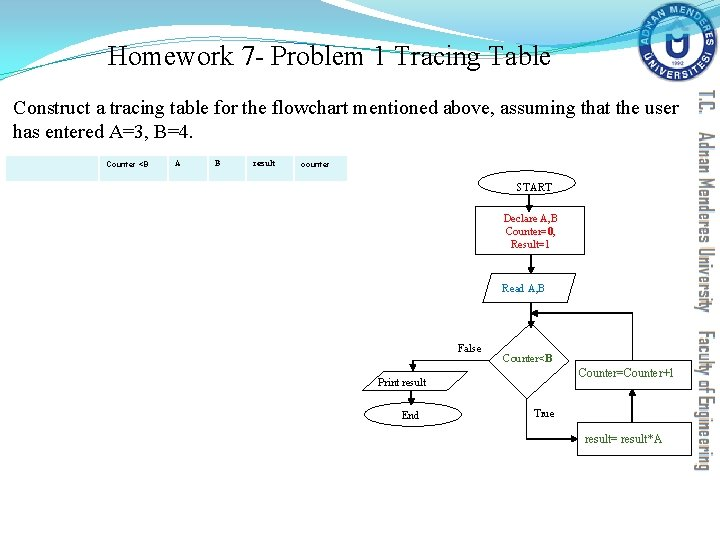Homework 7 - Problem 1 Tracing Table Construct a tracing table for the flowchart mentioned above, assuming that the user has entered A=3, B=4. Counter <B A B result counter START Declare A, B Counter=0, Result=1 Read A, B False Counter<B Counter=Counter+1 Print result End True result= result*A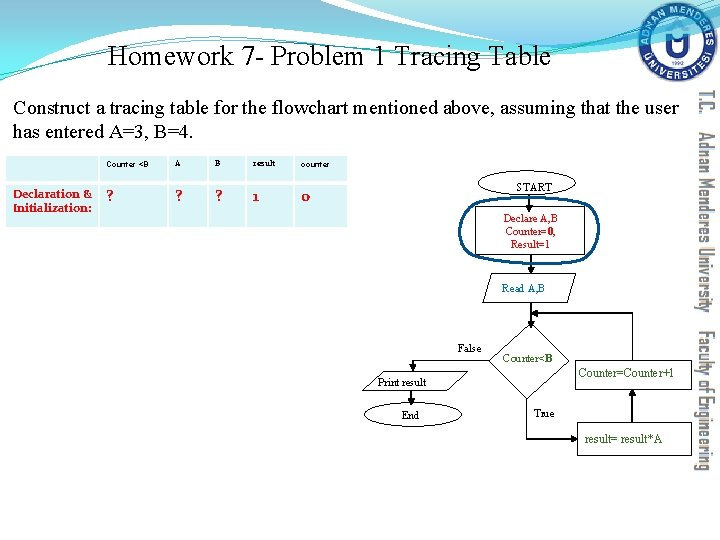Homework 7 - Problem 1 Tracing Table Construct a tracing table for the flowchart mentioned above, assuming that the user has entered A=3, B=4. Declaration & Initialization: Counter <B A B result counter ? ? ? 1 0 START Declare A, B Counter=0, Result=1 Read A, B False Counter<B Counter=Counter+1 Print result End True result= result*A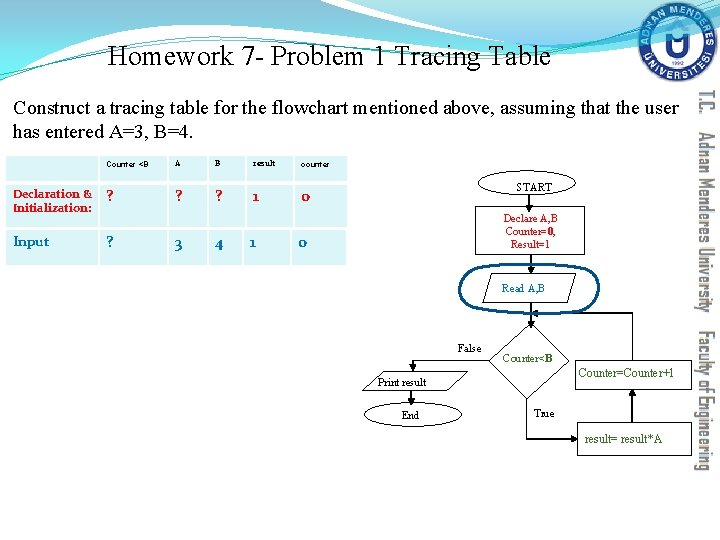Homework 7 - Problem 1 Tracing Table Construct a tracing table for the flowchart mentioned above, assuming that the user has entered A=3, B=4. Counter <B A B result counter Declaration & Initialization: ? ? ? 1 0 Input ? 3 4 1 START Declare A, B Counter=0, Result=1 0 Read A, B False Counter<B Counter=Counter+1 Print result End True result= result*A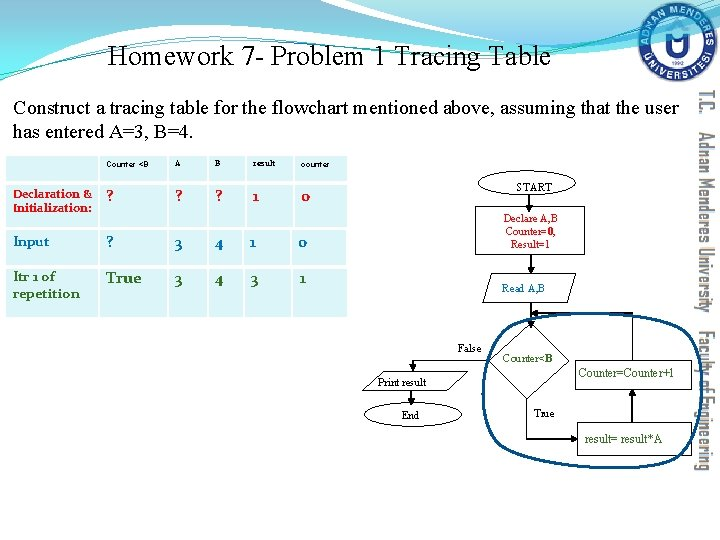Homework 7 - Problem 1 Tracing Table Construct a tracing table for the flowchart mentioned above, assuming that the user has entered A=3, B=4. Counter <B A B result counter Declaration & Initialization: ? ? ? 1 0 Input ? 3 4 1 0 Itr 1 of repetition True 3 4 3 1 START Declare A, B Counter=0, Result=1 Read A, B False Counter<B Counter=Counter+1 Print result End True result= result*A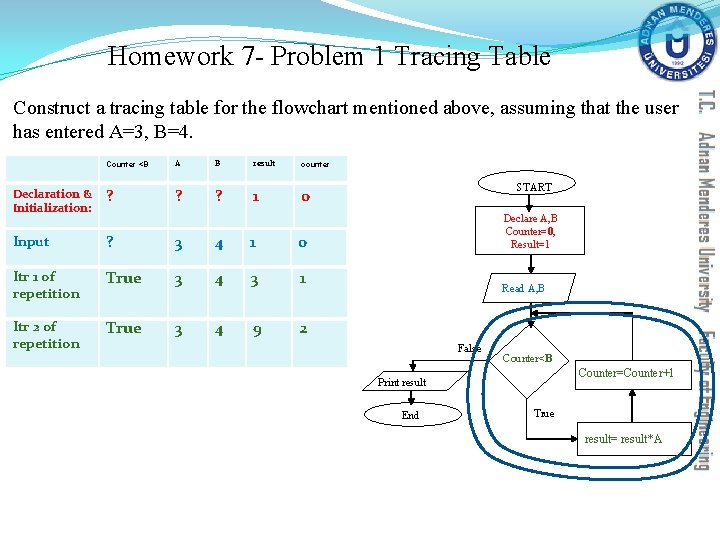Homework 7 - Problem 1 Tracing Table Construct a tracing table for the flowchart mentioned above, assuming that the user has entered A=3, B=4. Counter <B A B result counter Declaration & Initialization: ? ? ? 1 0 Input ? 3 4 1 0 Itr 1 of repetition True 3 4 3 1 Itr 2 of repetition True 3 4 9 2 START Declare A, B Counter=0, Result=1 Read A, B False Counter<B Counter=Counter+1 Print result End True result= result*A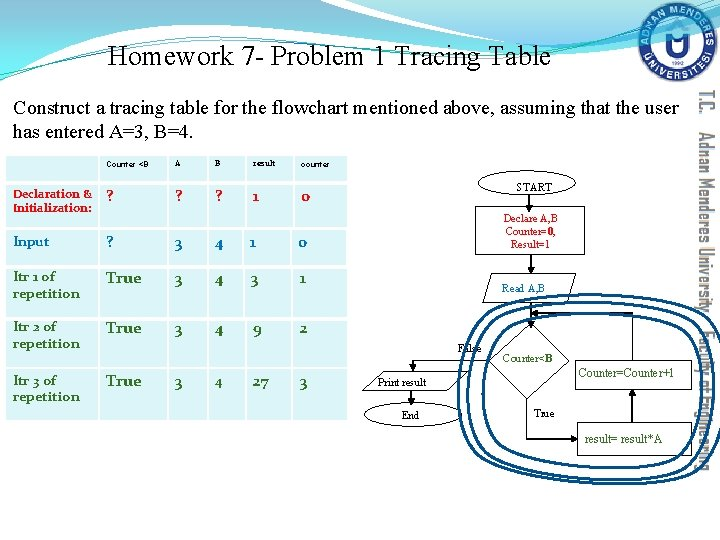Homework 7 - Problem 1 Tracing Table Construct a tracing table for the flowchart mentioned above, assuming that the user has entered A=3, B=4. Counter <B A B result counter Declaration & Initialization: ? ? ? 1 0 Input ? 3 4 1 0 Itr 1 of repetition True 3 4 3 1 Itr 2 of repetition True 3 4 9 2 Itr 3 of repetition START Declare A, B Counter=0, Result=1 Read A, B False True 3 4 27 3 Counter<B Counter=Counter+1 Print result End True result= result*A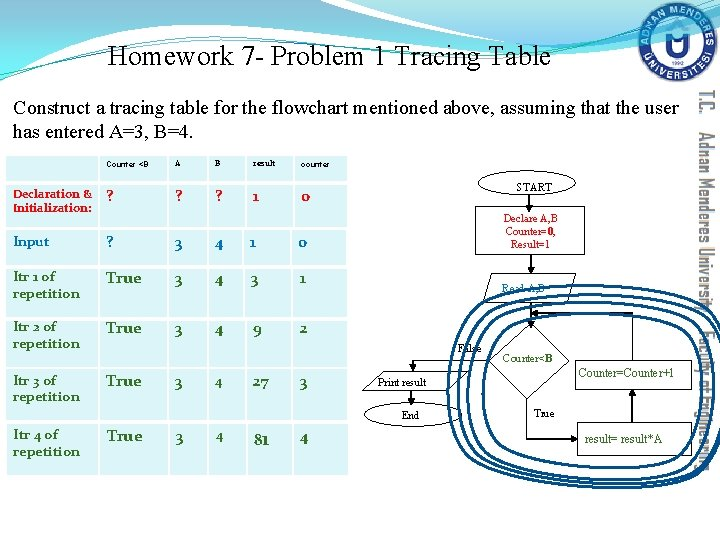Homework 7 - Problem 1 Tracing Table Construct a tracing table for the flowchart mentioned above, assuming that the user has entered A=3, B=4. Counter <B A B result counter Declaration & Initialization: ? ? ? 1 0 Input ? 3 4 1 0 Itr 1 of repetition True 3 4 3 1 Itr 2 of repetition True 3 4 9 2 Itr 3 of repetition START Declare A, B Counter=0, Result=1 Read A, B False True 3 4 27 3 True 3 4 81 4 Counter<B Counter=Counter+1 Print result End Itr 4 of repetition True result= result*AHomework 7 - Problem 1 Tracing Table Construct a tracing table for the flowchart mentioned above, assuming that the user has entered A=3, B=4. Counter <B A B result counter Declaration & Initialization: ? ? ? 1 0 Input ? 3 4 1 0 Itr 1 of repetition True 3 4 3 1 Itr 2 of repetition True 3 4 9 2 Itr 3 of repetition START Declare A, B Counter=0, Result=1 Read A, B False True 3 4 27 3 True 3 4 81 4 End of repetition & output false 3 4 81 4 Counter<B Counter=Counter+1 Print result End Itr 4 of repetition True result= result*A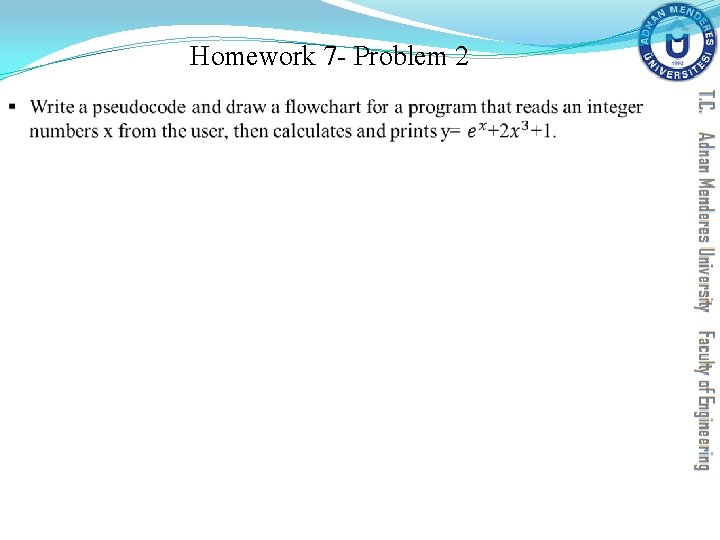Homework 7 - Problem 2 §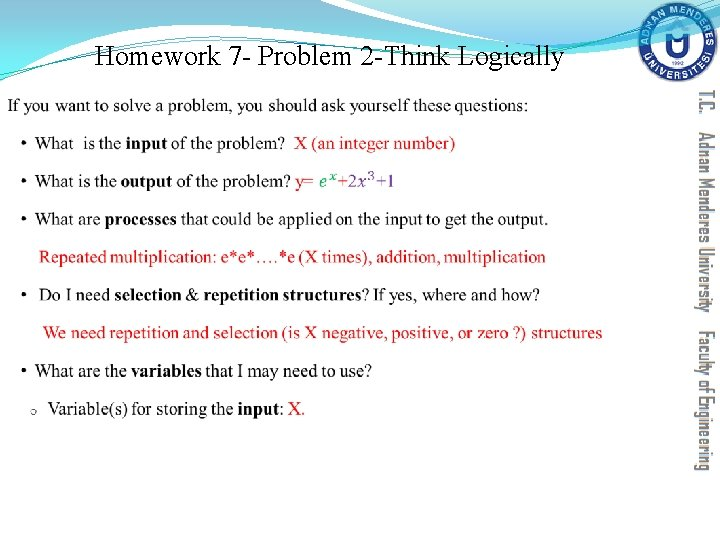Homework 7 - Problem 2 -Think Logically §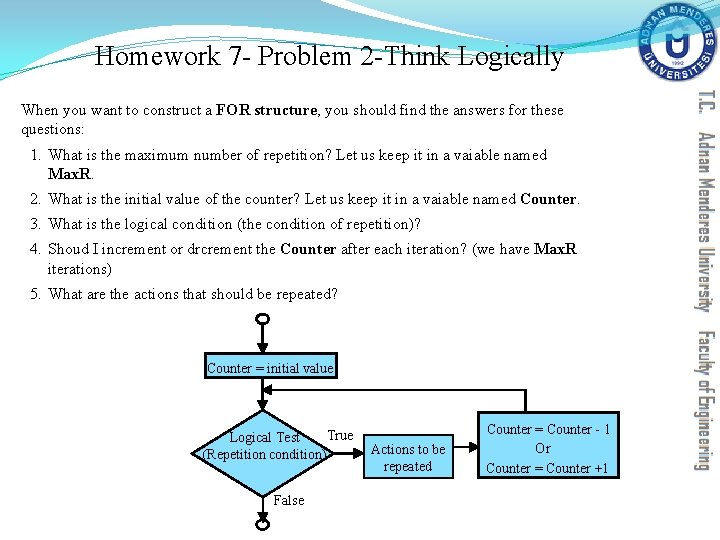Homework 7 - Problem 2 -Think Logically When you want to construct a FOR structure, you should find the answers for these questions: 1. What is the maximum number of repetition? Let us keep it in a vaiable named Max. R. 2. What is the initial value of the counter? Let us keep it in a vaiable named Counter. 3. What is the logical condition (the condition of repetition)? 4. Shoud I increment or drcrement the Counter after each iteration? (we have Max. R iterations) 5. What are the actions that should be repeated? Counter = initial value True Logical Test (Repetition condition) False Actions to be repeated Counter = Counter - 1 Or Counter = Counter +1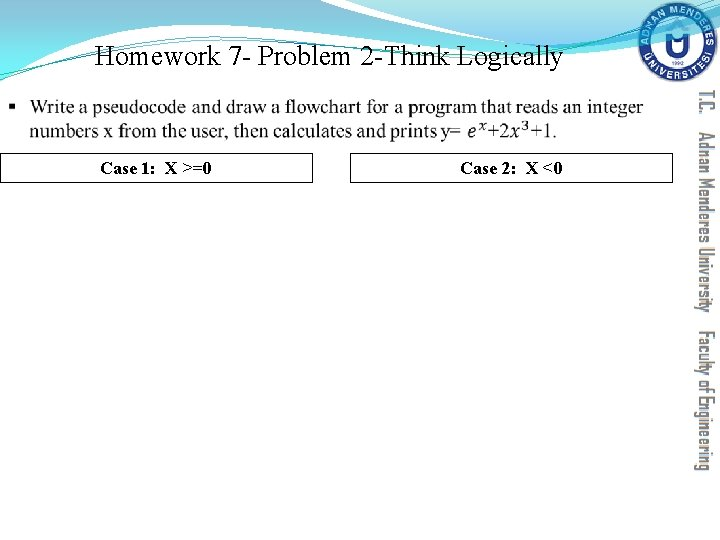Homework 7 - Problem 2 -Think Logically § Case 1: X >=0 Case 2: X <0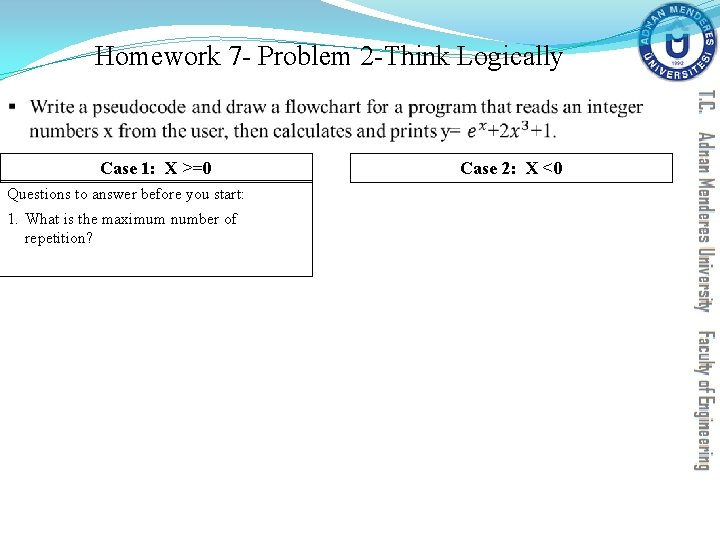Homework 7 - Problem 2 -Think Logically § Case 1: X >=0 Questions to answer before you start: 1. What is the maximum number of repetition? Case 2: X <0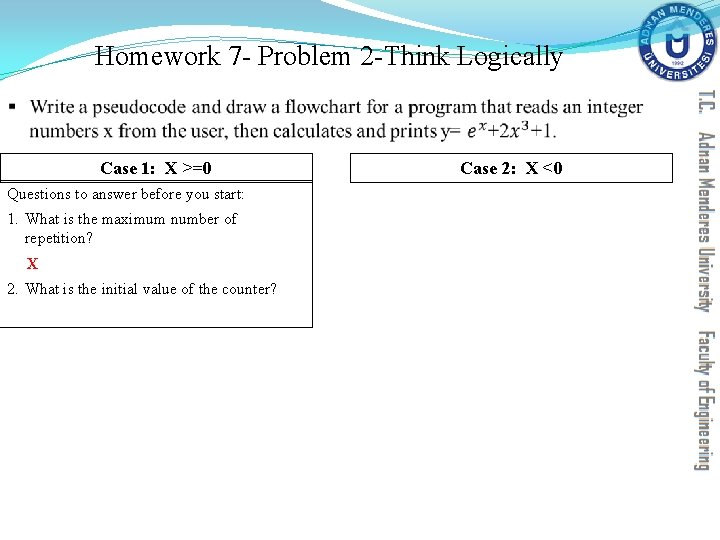Homework 7 - Problem 2 -Think Logically § Case 1: X >=0 Questions to answer before you start: 1. What is the maximum number of repetition? X 2. What is the initial value of the counter? Case 2: X <0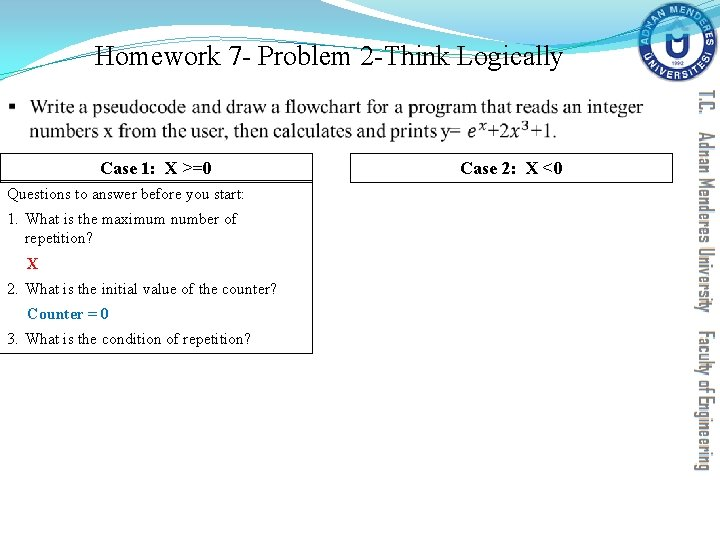Homework 7 - Problem 2 -Think Logically § Case 1: X >=0 Questions to answer before you start: 1. What is the maximum number of repetition? X 2. What is the initial value of the counter? Counter = 0 3. What is the condition of repetition? Case 2: X <0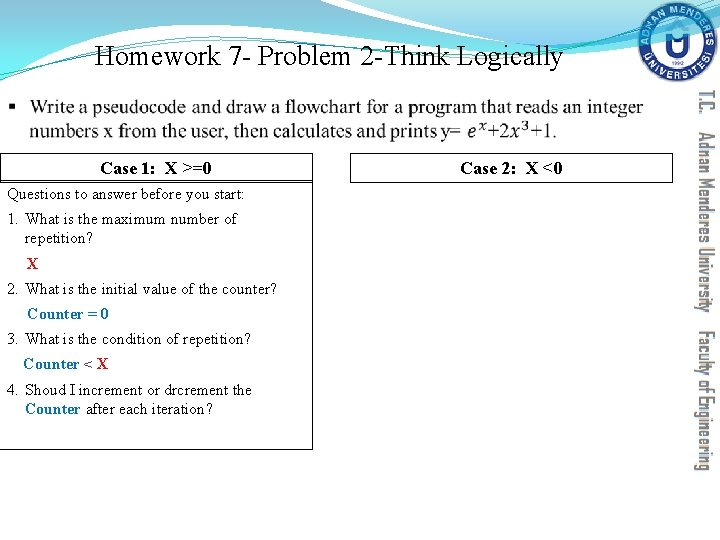Homework 7 - Problem 2 -Think Logically § Case 1: X >=0 Questions to answer before you start: 1. What is the maximum number of repetition? X 2. What is the initial value of the counter? Counter = 0 3. What is the condition of repetition? Counter < X 4. Shoud I increment or drcrement the Counter after each iteration? Case 2: X <0Homework 7 - Problem 2 -Think Logically § Case 1: X >=0 Questions to answer before you start: 1. What is the maximum number of repetition? X 2. What is the initial value of the counter? Counter = 0 3. What is the condition of repetition? Counter < X 4. Shoud I increment or drcrement the Counter after each iteration? Counter = Counter+1 5. What are the actions that should be repeated? Case 2: X <0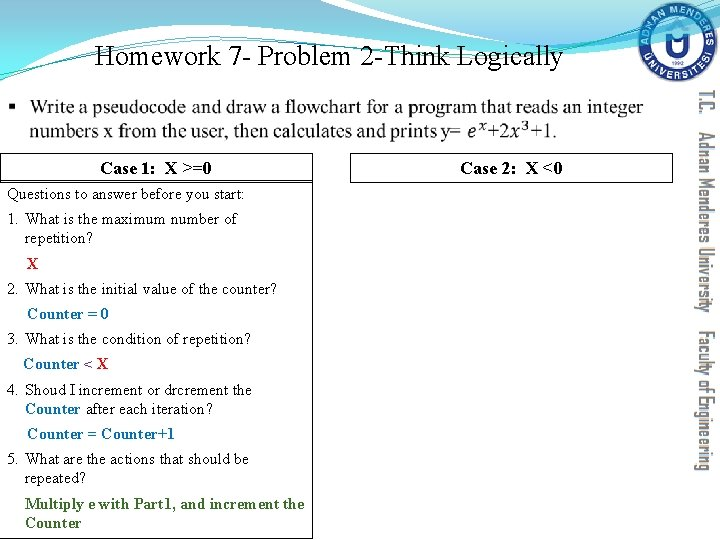Homework 7 - Problem 2 -Think Logically § Case 1: X >=0 Questions to answer before you start: 1. What is the maximum number of repetition? X 2. What is the initial value of the counter? Counter = 0 3. What is the condition of repetition? Counter < X 4. Shoud I increment or drcrement the Counter after each iteration? Counter = Counter+1 5. What are the actions that should be repeated? Multiply e with Part 1, and increment the Counter Case 2: X <0Homework 7 - Problem 2 -Think Logically § Case 1: X >=0 Case 2: X <0 Questions to answer before you start: 1. What is the maximum number of repetition? X 2. What is the initial value of the counter? Counter = 0 3. What is the condition of repetition? Counter < X 4. Shoud I increment or drcrement the Counter after each iteration? Counter = Counter+1 5. What are the actions that should be repeated? Multiply e with Part 1, and increment the Counter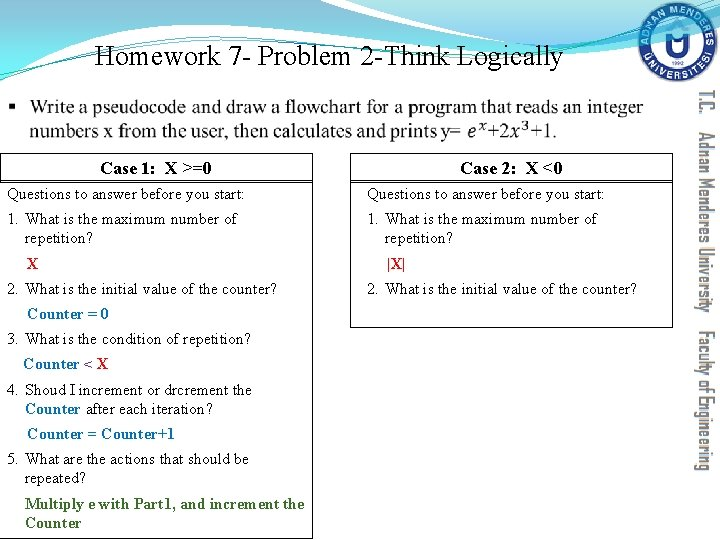Homework 7 - Problem 2 -Think Logically § Case 1: X >=0 Case 2: X <0 Questions to answer before you start: 1. What is the maximum number of repetition? X 2. What is the initial value of the counter? Counter = 0 3. What is the condition of repetition? Counter < X 4. Shoud I increment or drcrement the Counter after each iteration? Counter = Counter+1 5. What are the actions that should be repeated? Multiply e with Part 1, and increment the Counter |X| 2. What is the initial value of the counter?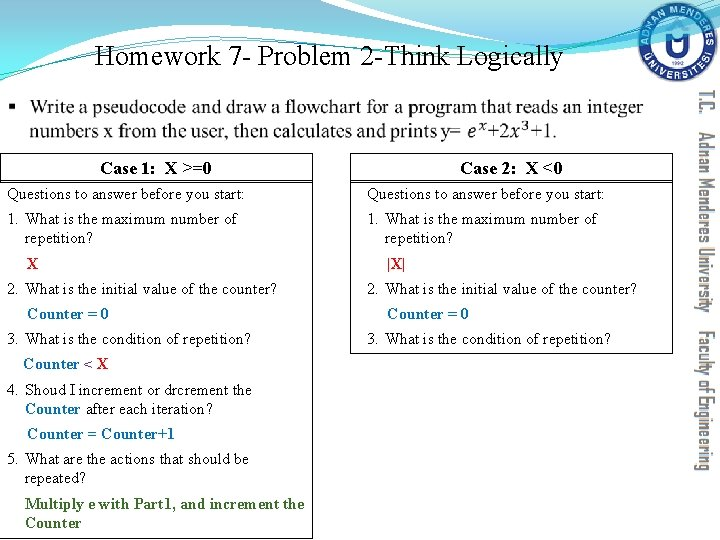Homework 7 - Problem 2 -Think Logically § Case 1: X >=0 Case 2: X <0 Questions to answer before you start: 1. What is the maximum number of repetition? X 2. What is the initial value of the counter? Counter = 0 3. What is the condition of repetition? Counter < X 4. Shoud I increment or drcrement the Counter after each iteration? Counter = Counter+1 5. What are the actions that should be repeated? Multiply e with Part 1, and increment the Counter |X| 2. What is the initial value of the counter? Counter = 0 3. What is the condition of repetition?Homework 7 - Problem 2 -Think Logically § Case 1: X >=0 Case 2: X <0 Questions to answer before you start: 1. What is the maximum number of repetition? X 2. What is the initial value of the counter? Counter = 0 3. What is the condition of repetition? Counter < X 4. Shoud I increment or drcrement the Counter after each iteration? Counter = Counter+1 5. What are the actions that should be repeated? Multiply e with Part 1, and increment the Counter |X| 2. What is the initial value of the counter? Counter = 0 3. What is the condition of repetition? Counter > X 4. Shoud I increment or drcrement the Counter after each iteration?Homework 7 - Problem 2 -Think Logically § Case 1: X >=0 Case 2: X <0 Questions to answer before you start: 1. What is the maximum number of repetition? X 2. What is the initial value of the counter? Counter = 0 3. What is the condition of repetition? Counter < X 4. Shoud I increment or drcrement the Counter after each iteration? Counter = Counter+1 5. What are the actions that should be repeated? Multiply e with Part 1, and increment the Counter |X| 2. What is the initial value of the counter? Counter = 0 3. What is the condition of repetition? Counter > X 4. Shoud I increment or drcrement the Counter after each iteration? Counter = Counter-1 5. What are the actions that should be repeated?Homework 7 - Problem 2 -Think Logically § Case 1: X >=0 Case 2: X <0 Questions to answer before you start: 1. What is the maximum number of repetition? X 2. What is the initial value of the counter? Counter = 0 3. What is the condition of repetition? Counter < X 4. Shoud I increment or drcrement the Counter after each iteration? Counter = Counter+1 5. What are the actions that should be repeated? Multiply e with Part 1, and increment the Counter |X| 2. What is the initial value of the counter? Counter = 0 3. What is the condition of repetition? Counter > X 4. Shoud I increment or drcrement the Counter after each iteration? Counter = Counter-1 5. What are the actions that should be repeated? Divide Part 1 over e, and decrement the CounterHomework 7 - Problem 2 Pseudocode § Pseudocode: Declare X, Counter, Part 1=1, Part 2, Result Read X IF (X<0) THEN ELSE ENDIFHomework 7 - Problem 2 Pseudocode § Pseudocode: Declare X, Counter, Part 1=1, Part 2, Result Read X IF (X<0) THEN FOR(Counter=0; Counter>X; Counter=Counter-1) Part 1=Part 1/e ENDFOR ELSE ENDIF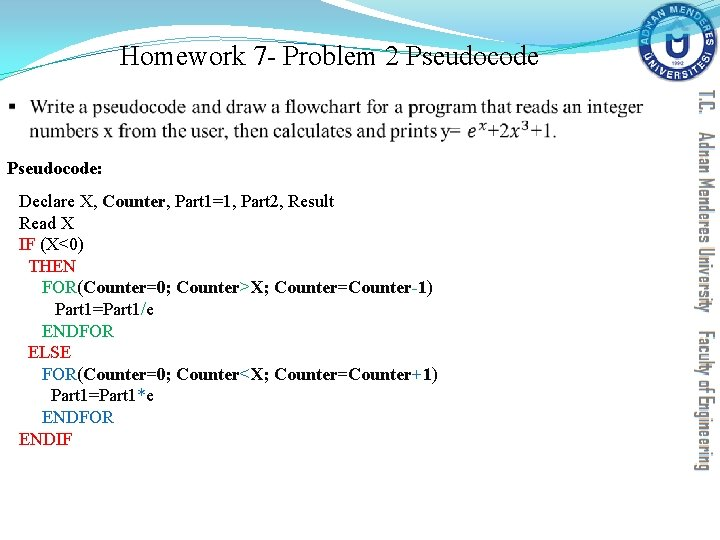Homework 7 - Problem 2 Pseudocode § Pseudocode: Declare X, Counter, Part 1=1, Part 2, Result Read X IF (X<0) THEN FOR(Counter=0; Counter>X; Counter=Counter-1) Part 1=Part 1/e ENDFOR ELSE FOR(Counter=0; Counter<X; Counter=Counter+1) Part 1=Part 1*e ENDFOR ENDIFHomework 7 - Problem 2 Pseudocode § Pseudocode: Declare X, Counter, Part 1=1, Part 2, Result Read X IF (X<0) THEN FOR(Counter=0; Counter>X; Counter=Counter-1) Part 1=Part 1/e ENDFOR ELSE FOR(Counter=0; Counter<X; Counter=Counter+1) Part 1=Part 1*e ENDFOR ENDIF Part 2=2*X*X*X+1 Result=Part 1+Part 2 Write ResultHomework 7 - Problem 2 Flowchart Declare X, Counter, Part 1=1, Part 2, Result Read X START Declare X, Counter=0, Part 1=1, Part 2, Result IF (X<0) THEN FOR(Counter=0; Counter>X; Counter=Counter-1) Part 1=Part 1/e ENDFOR ELSE FOR(Counter=0; Counter<X; Counter=Counter+1) Part 1=Part 1*e ENDFOR ENDIF Part 2=2*X*X*X+1 Result=Part 1+Part 2 Write Result Read X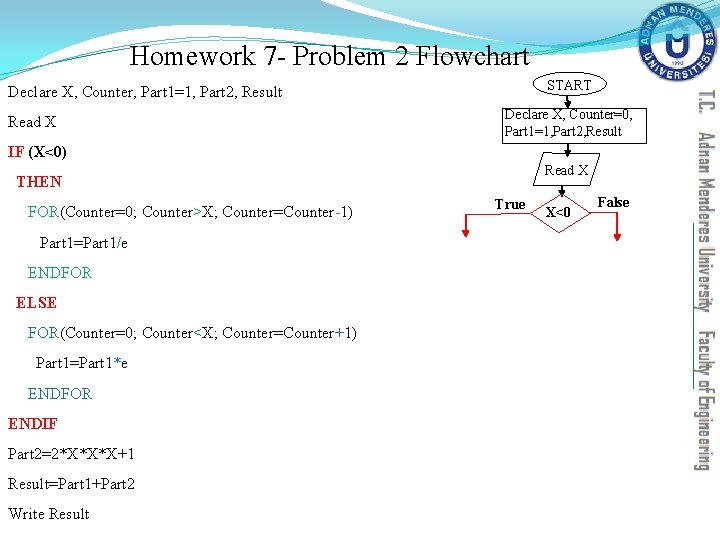Homework 7 - Problem 2 Flowchart START Declare X, Counter, Part 1=1, Part 2, Result Read X Declare X, Counter=0, Part 1=1, Part 2, Result IF (X<0) Read X THEN FOR(Counter=0; Counter>X; Counter=Counter-1) Part 1=Part 1/e ENDFOR ELSE FOR(Counter=0; Counter<X; Counter=Counter+1) Part 1=Part 1*e ENDFOR ENDIF Part 2=2*X*X*X+1 Result=Part 1+Part 2 Write Result True X<0 FalseHomework 7 - Problem 2 Flowchart START Declare X, Counter, Part 1=1, Part 2, Result Declare X, Counter=0, Part 1=1, Part 2, Result Read X IF (X<0) Read X THEN True FOR(Counter=0; Counter>X; Counter=Counter-1) Part 1=Part 1/e ENDFOR ELSE Counter>X True Part 1= Part 1/e FOR(Counter=0; Counter<X; Counter=Counter+1) Part 1=Part 1*e ENDFOR ENDIF Part 2=2*X*X*X+1 Result=Part 1+Part 2 Write Result Counter=Counter-1 X<0 False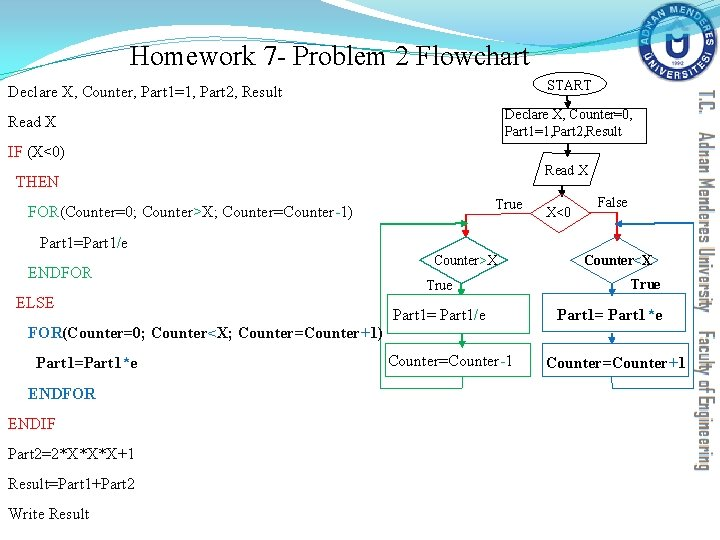Homework 7 - Problem 2 Flowchart START Declare X, Counter, Part 1=1, Part 2, Result Declare X, Counter=0, Part 1=1, Part 2, Result Read X IF (X<0) Read X THEN True FOR(Counter=0; Counter>X; Counter=Counter-1) ELSE False Part 1=Part 1/e ENDFOR X<0 Counter>X True Part 1= Part 1/e Counter<X True Part 1= Part 1*e FOR(Counter=0; Counter<X; Counter=Counter+1) Part 1=Part 1*e ENDFOR ENDIF Part 2=2*X*X*X+1 Result=Part 1+Part 2 Write Result Counter=Counter-1 Counter=Counter+1Homework 7 - Problem 2 Flowchart START Declare X, Counter, Part 1=1, Part 2, Result Declare X, Counter=0, Part 1=1, Part 2, Result Read X IF (X<0) Read X THEN True FOR(Counter=0; Counter>X; Counter=Counter-1) Part 1=Part 1/e ENDFOR ELSE Counter>X False X<0 False Counter<X True Part 1= Part 1/e Part 1= Part 1*e FOR(Counter=0; Counter<X; Counter=Counter+1) Part 1=Part 1*e Counter=Counter-1 Counter=Counter+1 ENDFOR ENDIF Part 2= 2*X*X*X+1 Part 2=2*X*X*X+1 Result=Part 1+Part 2 Print result Write Result ENDHomework 7 - Problem 2 START § Does this flowchart represent another correct method for solving problem 2? Declare X, Flag=0 Counter=0, Part 1=1, Part 2, Result Read X True X=-1*X Flag=1 False Part 2= 2*X*X*X+1 True Flag==1 X<0 False Counter<X True Counter=Counter+1 False Part 1= Part 1*e Part 1=1/Part 1 result=Part 1+Part 2 Print result EndHomework 7 - Problem 2 START § Write an equivalent pseudocode for it. Declare X, Flag=0 Counter=0, Part 1=1, Part 2, Result Read X True X=-1*X Flag=1 False Part 2= 2*X*X*X+1 True Flag==1 X<0 False Counter<X True Counter=Counter+1 False Part 1= Part 1*e Part 1=1/Part 1 result=Part 1+Part 2 Print result End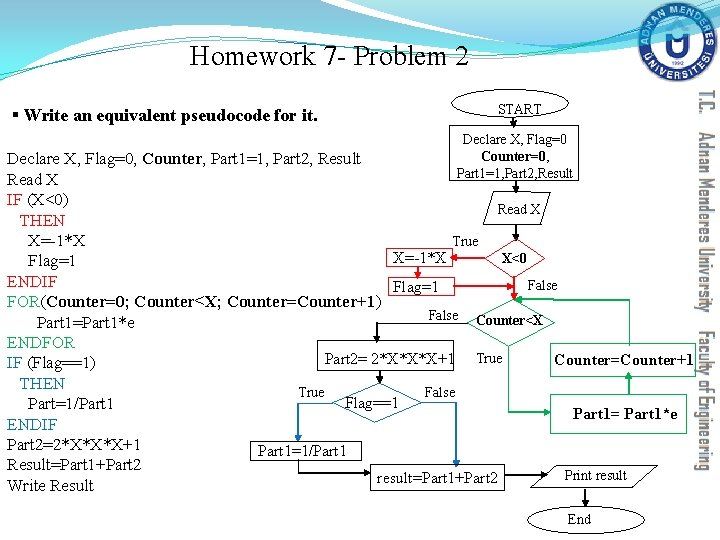Homework 7 - Problem 2 § Write an equivalent pseudocode for it. START Declare X, Flag=0 Counter=0, Part 1=1, Part 2, Result Declare X, Flag=0, Counter, Part 1=1, Part 2, Result Read X IF (X<0) Read X THEN True X=-1*X X<0 X=-1*X Flag=1 ENDIF False Flag=1 FOR(Counter=0; Counter<X; Counter=Counter+1) False Counter<X Part 1=Part 1*e ENDFOR Part 2= 2*X*X*X+1 True Counter=Counter+1 IF (Flag==1) THEN True False Flag==1 Part=1/Part 1= Part 1*e ENDIF Part 2=2*X*X*X+1 Part 1=1/Part 1 Result=Part 1+Part 2 Print result=Part 1+Part 2 Write Result End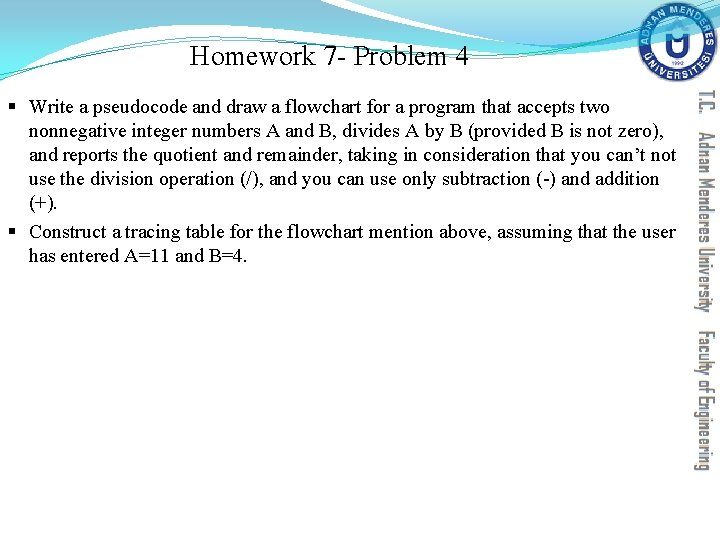Homework 7 - Problem 4 § Write a pseudocode and draw a flowchart for a program that accepts two nonnegative integer numbers A and B, divides A by B (provided B is not zero), and reports the quotient and remainder, taking in consideration that you can’t not use the division operation (/), and you can use only subtraction (-) and addition (+). § Construct a tracing table for the flowchart mention above, assuming that the user has entered A=11 and B=4.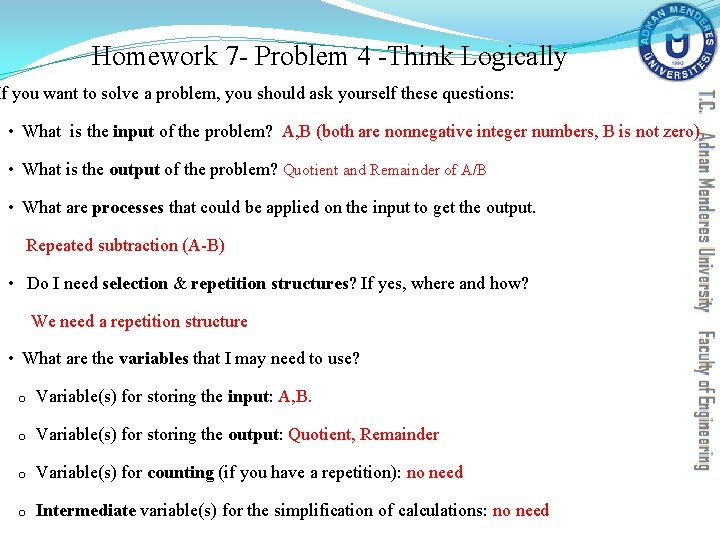Homework 7 - Problem 4 -Think Logically If you want to solve a problem, you should ask yourself these questions: • What is the input of the problem? A, B (both are nonnegative integer numbers, B is not zero) • What is the output of the problem? Quotient and Remainder of A/B • What are processes that could be applied on the input to get the output. Repeated subtraction (A-B) • Do I need selection & repetition structures? If yes, where and how? We need a repetition structure • What are the variables that I may need to use? o Variable(s) for storing the input: A, B. o Variable(s) for storing the output: Quotient, Remainder o Variable(s) for counting (if you have a repetition): no need o Intermediate variable(s) for the simplification of calculations: no needHomework 7 - Problem 4 -Think Logically When you want to construct a FOR structure, you should find the answers for these questions: 1. What is the maximum number of repetition? Let us keep it in a vaiable named Max. R. 2. What is the initial value of the counter? Let us keep it in a vaiable named Counter. 3. What is the logical condition (the condition of repetition)? 4. Shoud I increment or drcrement the Counter after each iteration? (we have Max. R iterations) 5. What are the actions that should be repeated? Counter = initial value True Logical Test (Repetition condition) False Actions to be repeated Counter = Counter - 1 Or Counter = Counter +1Homework 7 - Problem 4 -Think Logically § Write a pseudocode and draw a flowchart for a program that accepts two nonnegative integer numbers A and B, divides A by B (provided B is not zero), and reports the quotient and remainder, taking in consideration that you can’t not use the division operation (/), and you can use only subtraction (-) and addition (+). Questions to answer before you start: 1. What is the maximum number of repetition?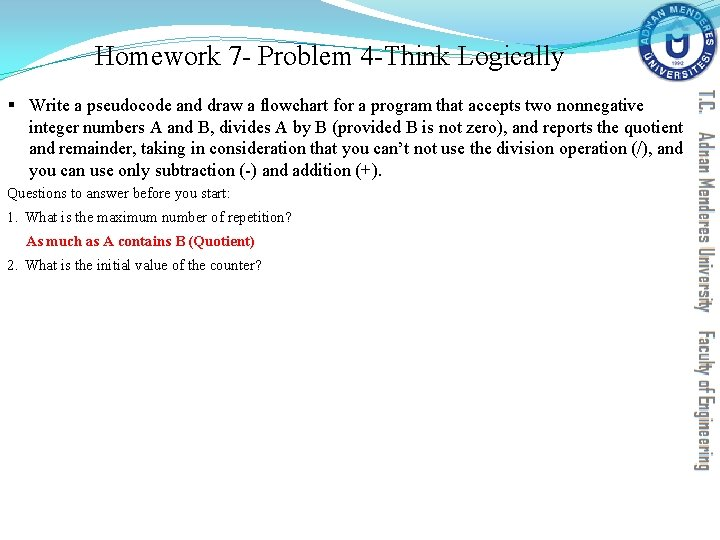Homework 7 - Problem 4 -Think Logically § Write a pseudocode and draw a flowchart for a program that accepts two nonnegative integer numbers A and B, divides A by B (provided B is not zero), and reports the quotient and remainder, taking in consideration that you can’t not use the division operation (/), and you can use only subtraction (-) and addition (+). Questions to answer before you start: 1. What is the maximum number of repetition? As much as A contains B (Quotient) 2. What is the initial value of the counter?Homework 7 - Problem 4 -Think Logically § Write a pseudocode and draw a flowchart for a program that accepts two nonnegative integer numbers A and B, divides A by B (provided B is not zero), and reports the quotient and remainder, taking in consideration that you can’t not use the division operation (/), and you can use only subtraction (-) and addition (+). Questions to answer before you start: 1. What is the maximum number of repetition? As much as A contains B (Quotient) 2. What is the initial value of the counter? There no explicit variable for counting 3. What is the condition of repetition?Homework 7 - Problem 4 -Think Logically § Write a pseudocode and draw a flowchart for a program that accepts two nonnegative integer numbers A and B, divides A by B (provided B is not zero), and reports the quotient and remainder, taking in consideration that you can’t not use the division operation (/), and you can use only subtraction (-) and addition (+). Questions to answer before you start: 1. What is the maximum number of repetition? As much as A contains B (Quotient) 2. What is the initial value of the counter? There no explicit variable for counting 3. What is the condition of repetition? A>=B 4. Shoud I increment or drcrement the Counter after each iteration?Homework 7 - Problem 4 -Think Logically § Write a pseudocode and draw a flowchart for a program that accepts two nonnegative integer numbers A and B, divides A by B (provided B is not zero), and reports the quotient and remainder, taking in consideration that you can’t not use the division operation (/), and you can use only subtraction (-) and addition (+). Questions to answer before you start: 1. What is the maximum number of repetition? As much as A contains B (Quotient) 2. What is the initial value of the counter? There no explicit variable for counting 3. What is the condition of repetition? A>=B 4. Shoud I increment or drcrement the Counter after each iteration? There no explicit variable for counting 5. What are the actions that should be repeated?Homework 7 - Problem 4 -Think Logically § Write a pseudocode and draw a flowchart for a program that accepts two nonnegative integer numbers A and B, divides A by B (provided B is not zero), and reports the quotient and remainder, taking in consideration that you can’t not use the division operation (/), and you can use only subtraction (-) and addition (+). Questions to answer before you start: 1. What is the maximum number of repetition? As much as A contains B (Quotient) 2. What is the initial value of the counter? There no explicit variable for counting 3. What is the condition of repetition? A>=B 4. Shoud I increment or drcrement the Counter after each iteration? There no explicit variable for counting 5. What are the actions that should be repeated? Subtracting B from A, Increasing quotient by 1,Homework 7 - Problem 4 Pseudocode § Write a pseudocode and draw a flowchart for a program that accepts two nonnegative integer numbers A and B, divides A by B (provided B is not zero), and reports the quotient and remainder, taking in consideration that you can’t not use the division operation (/), and you can use only subtraction (-) and addition (+). Questions to answer before you start: Psudocode: 1. What is the maximum number of repetition? Declare A, B, Quotient=0, Remainder As much as A contains B (Quotient) 2. What is the initial value of the counter? There no explicit variable for counting 3. What is the condition of repetition? A>=B 4. Shoud I increment or drcrement the Counter after each iteration? There no explicit variable for counting 5. What are the actions that should be repeated? Subtracting B from A, Increasing quotient by 1, Read A, BHomework 7 - Problem 4 Pseudocode § Write a pseudocode and draw a flowchart for a program that accepts two nonnegative integer numbers A and B, divides A by B (provided B is not zero), and reports the quotient and remainder, taking in consideration that you can’t not use the division operation (/), and you can use only subtraction (-) and addition (+). Questions to answer before you start: Psudocode: 1. What is the maximum number of repetition? Declare A, B, Quotient=0, Remainder As much as A contains B (Quotient) 2. What is the initial value of the counter? There no explicit variable for counting 3. What is the condition of repetition? A>=B 4. Shoud I increment or drcrement the Counter after each iteration? There no explicit variable for counting 5. What are the actions that should be repeated? Subtracting B from A, Increasing quotient by 1, Read A, B WHILE (Condition of Repetition ) Actions that should be repeated ENDWHILE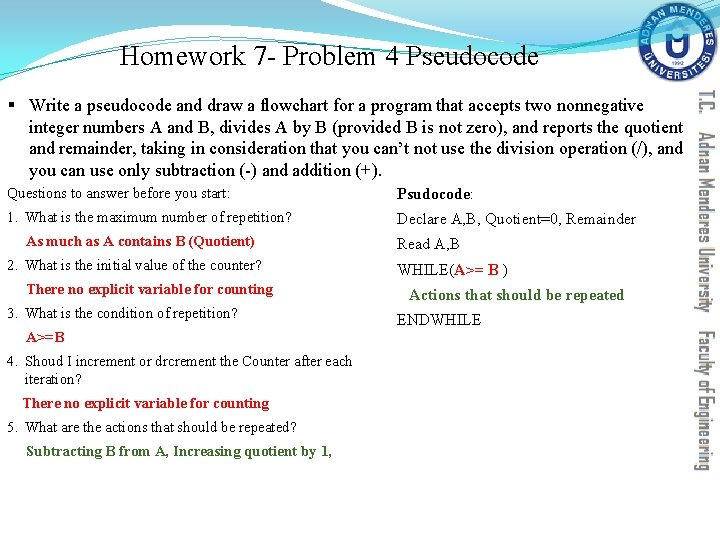Homework 7 - Problem 4 Pseudocode § Write a pseudocode and draw a flowchart for a program that accepts two nonnegative integer numbers A and B, divides A by B (provided B is not zero), and reports the quotient and remainder, taking in consideration that you can’t not use the division operation (/), and you can use only subtraction (-) and addition (+). Questions to answer before you start: Psudocode: 1. What is the maximum number of repetition? Declare A, B, Quotient=0, Remainder As much as A contains B (Quotient) 2. What is the initial value of the counter? There no explicit variable for counting 3. What is the condition of repetition? A>=B 4. Shoud I increment or drcrement the Counter after each iteration? There no explicit variable for counting 5. What are the actions that should be repeated? Subtracting B from A, Increasing quotient by 1, Read A, B WHILE(A>= B ) Actions that should be repeated ENDWHILE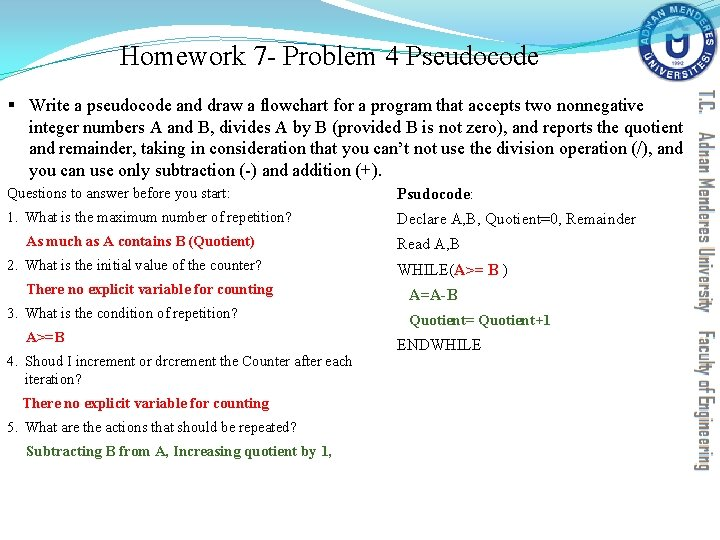Homework 7 - Problem 4 Pseudocode § Write a pseudocode and draw a flowchart for a program that accepts two nonnegative integer numbers A and B, divides A by B (provided B is not zero), and reports the quotient and remainder, taking in consideration that you can’t not use the division operation (/), and you can use only subtraction (-) and addition (+). Questions to answer before you start: Psudocode: 1. What is the maximum number of repetition? Declare A, B, Quotient=0, Remainder As much as A contains B (Quotient) 2. What is the initial value of the counter? There no explicit variable for counting 3. What is the condition of repetition? A>=B 4. Shoud I increment or drcrement the Counter after each iteration? There no explicit variable for counting 5. What are the actions that should be repeated? Subtracting B from A, Increasing quotient by 1, Read A, B WHILE(A>= B ) A=A-B Quotient= Quotient+1 ENDWHILEHomework 7 - Problem 4 Pseudocode § Write a pseudocode and draw a flowchart for a program that accepts two nonnegative integer numbers A and B, divides A by B (provided B is not zero), and reports the quotient and remainder, taking in consideration that you can’t not use the division operation (/), and you can use only subtraction (-) and addition (+). Questions to answer before you start: Psudocode: 1. What is the maximum number of repetition? Declare A, B, Quotient=0, Remainder As much as A contains B (Quotient) 2. What is the initial value of the counter? There no explicit variable for counting 3. What is the condition of repetition? A>=B 4. Shoud I increment or drcrement the Counter after each iteration? There no explicit variable for counting 5. What are the actions that should be repeated? Subtracting B from A, Increasing quotient by 1, Read A, B WHILE(A>= B ) A=A-B Quotient= Quotient+1 ENDWHILE Remainder=A Write Quotient, RemainderHomework 7 - Problem 4 Flowchart § Write a pseudocode and draw a flowchart for a program that accepts two nonnegative integer numbers A and B, divides A by B (provided B is not zero), and reports the quotient and remainder, taking in consideration that you can’t not use the division operation (/), and you can use only subtraction (-) and addition (+). start Psudocode: Declare A, B, Quotient=0, Remainder Read A, B WHILE(A>= B ) Declare A, B Quotient=0 Read A, B A=A-B Quotient= Quotient+1 Remainder=A No A ≥ B Write Quotient, Remainder Yes A=A-B Quotient= Quotient+1 Remainder =A Write Remainder, Quotient endHomework 7 - Problem 4 Tracing Table § Construct a tracing table for the flowchart mention above, assuming that the user has entered A=11 and B=4. A>=B A B Quotient Remainder start Declare A, B Quotient=0 Read A, B No A ≥ B Yes A=A-B Quotient= Quotient+1 Remainder =A Write Remainder, Quotient end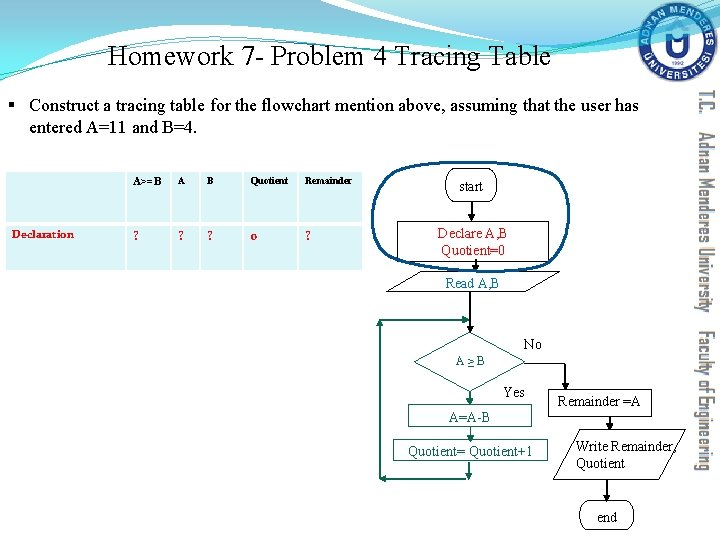Homework 7 - Problem 4 Tracing Table § Construct a tracing table for the flowchart mention above, assuming that the user has entered A=11 and B=4. Declaration A>=B A B Quotient Remainder ? ? ? 0 ? start Declare A, B Quotient=0 Read A, B No A ≥ B Yes A=A-B Quotient= Quotient+1 Remainder =A Write Remainder, Quotient endHomework 7 - Problem 4 Tracing Table § Construct a tracing table for the flowchart mention above, assuming that the user has entered A=11 and B=4. A>=B A B Quotient Remainder Declaration ? ? ? 0 ? Declare A, B Quotient=0 Input ? 11 4 0 ? Read A, B start No A ≥ B Yes A=A-B Quotient= Quotient+1 Remainder =A Write Remainder, Quotient end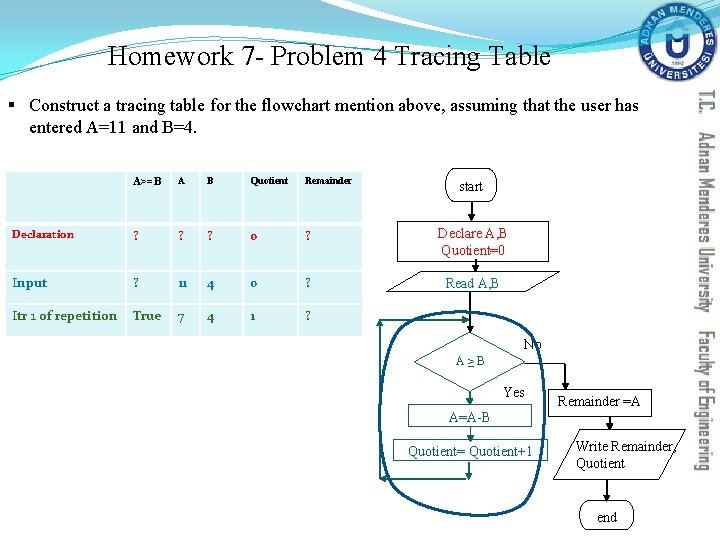Homework 7 - Problem 4 Tracing Table § Construct a tracing table for the flowchart mention above, assuming that the user has entered A=11 and B=4. A>=B A B Quotient Remainder Declaration ? ? ? 0 ? Declare A, B Quotient=0 Input ? 11 4 0 ? Read A, B Itr 1 of repetition True 7 4 1 ? start No A ≥ B Yes A=A-B Quotient= Quotient+1 Remainder =A Write Remainder, Quotient endHomework 7 - Problem 4 Tracing Table § Construct a tracing table for the flowchart mention above, assuming that the user has entered A=11 and B=4. A>=B A B Quotient Remainder Declaration ? ? ? 0 ? Declare A, B Quotient=0 Input ? 11 4 0 ? Read A, B Itr 1 of repetition True 7 4 1 ? Itr 2 of repetition True 3 4 2 ? start No A ≥ B Yes A=A-B Quotient= Quotient+1 Remainder =A Write Remainder, Quotient end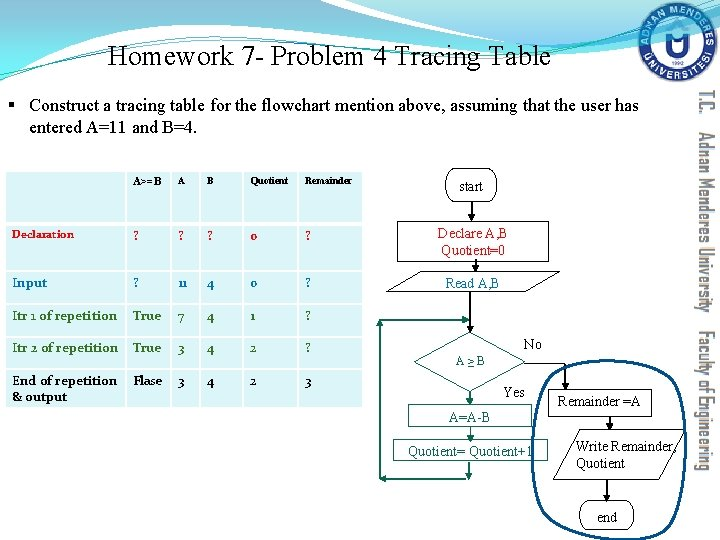Homework 7 - Problem 4 Tracing Table § Construct a tracing table for the flowchart mention above, assuming that the user has entered A=11 and B=4. A>=B A B Quotient Remainder Declaration ? ? ? 0 ? Declare A, B Quotient=0 Input ? 11 4 0 ? Read A, B Itr 1 of repetition True 7 4 1 ? Itr 2 of repetition True 3 4 2 ? End of repetition & output Flase 3 4 2 3 start No A ≥ B Yes A=A-B Quotient= Quotient+1 Remainder =A Write Remainder, Quotient endhttp: //humorhub. net/which-step-have-you-reached-today/